# SSAT Elementary Level Math : How to find a proportion

## Example Questions

← Previous 1

### Example Question #1 : How To Find A Proportion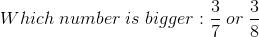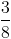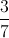Explanation: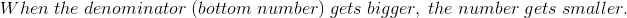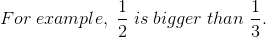### Example Question #2 : How To Find A Proportion

What is 10% of 100?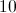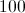Explanation:

10% of 100 is calculated by multiplying .10 by 100.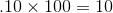### Example Question #3 : How To Find A Proportion

What is 13% of 100?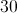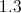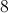Explanation:

13% of 100 is calculated by multiplying .13 by 100.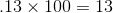### Example Question #4 : How To Find A Proportion

Solve for x: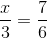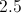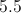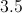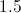Explanation:

Multiply both sides by 3 to yield: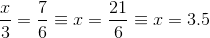### Example Question #5 : How To Find A Proportion

Brad is paid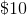per hour at his current job. If a promotion would increase Brad's pay by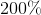, what would Brad make per hour after a promotion?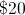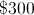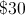per hour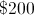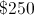per hour

Explanation:

To findof Brad's pay, multiply it by(since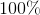is the original number andis twice that).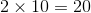, which is the amount of the increase to Brad's pay. Add this increase to the original pay to get the new pay: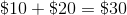per hour.

### Example Question #6 : How To Find A Proportion

Sofia goes to the movies with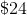. She spends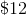on her ticket and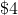on food and drink.

What fraction of Sofia's originalwas not spent?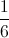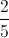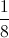Explanation:

Adding Sofia's expenses shows that she spent a total of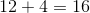dollars. Subtracting this from the original amount of money she had gives the unspent portion: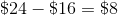The ratio of the unspent portion to the original amount gives the fraction of the money that was not spent: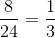.

### Example Question #21 : Ssat Elementary Level Quantitative (Math)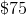sweater is on sale for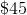. What percentage of the original price has been discounted for the sale?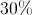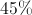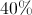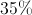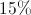Explanation:

Set up a proportion of the new price to the old price to see how they relate.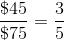of the original price

Subtractfromto find the amount that was discounted.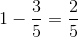Convertto a decimal by multiplying both the top and bottom of the fraction by, then convert to a percentage.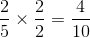, which is equivalent to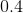or### Example Question #8 : How To Find A Proportion

Mary wants to enlarge a picture that she has in her office. The original picture iscm (width) bycm (length). What would be the length of the enlarged picture if the width iscm?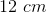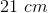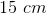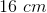Explanation:

To find the length of the enlarged picture, we must set up a proportion: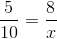cross multiply: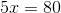Divide both sides by 5: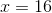### Example Question #9 : How To Find A Proportion

Solve for y: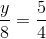Explanation:

Multiply both sides by 8 to yield: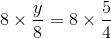Therefore: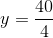Therefore: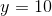### Example Question #10 : How To Find A Proportion

Solve for the variable: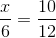15

8

4

3

5

5

Explanation:

Cross multiply: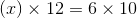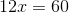Solve for x by dividing 60 by 12:← Previous 1